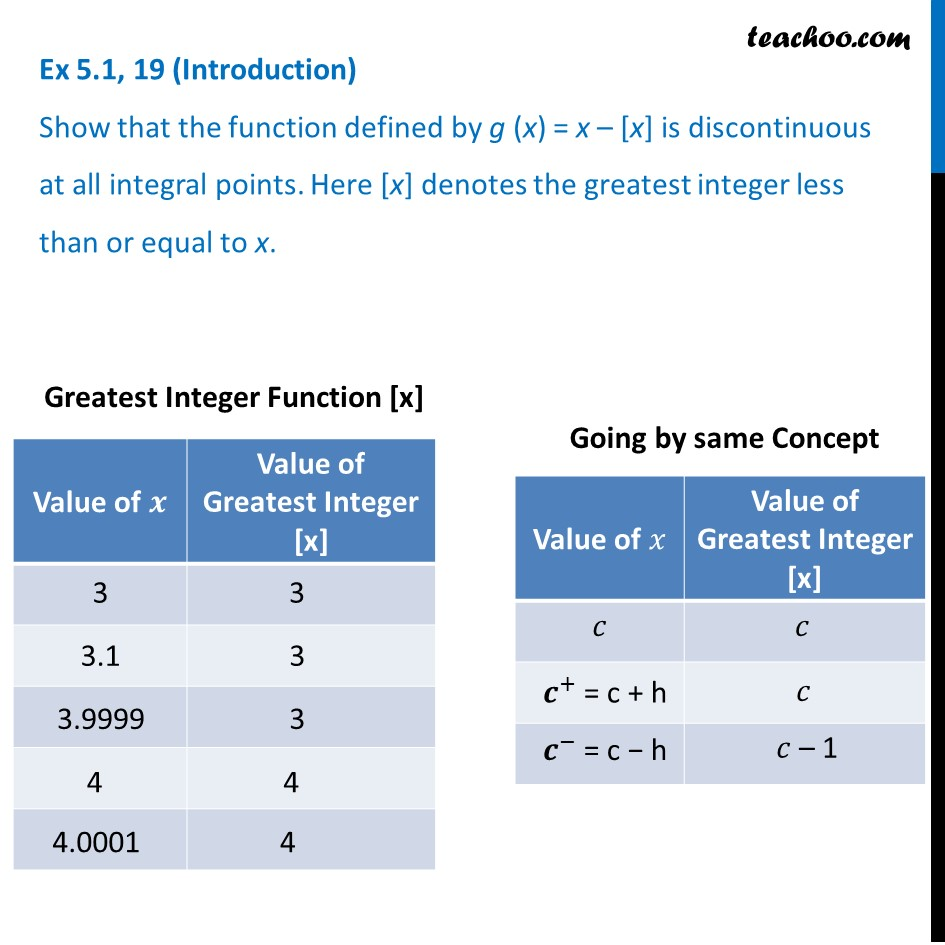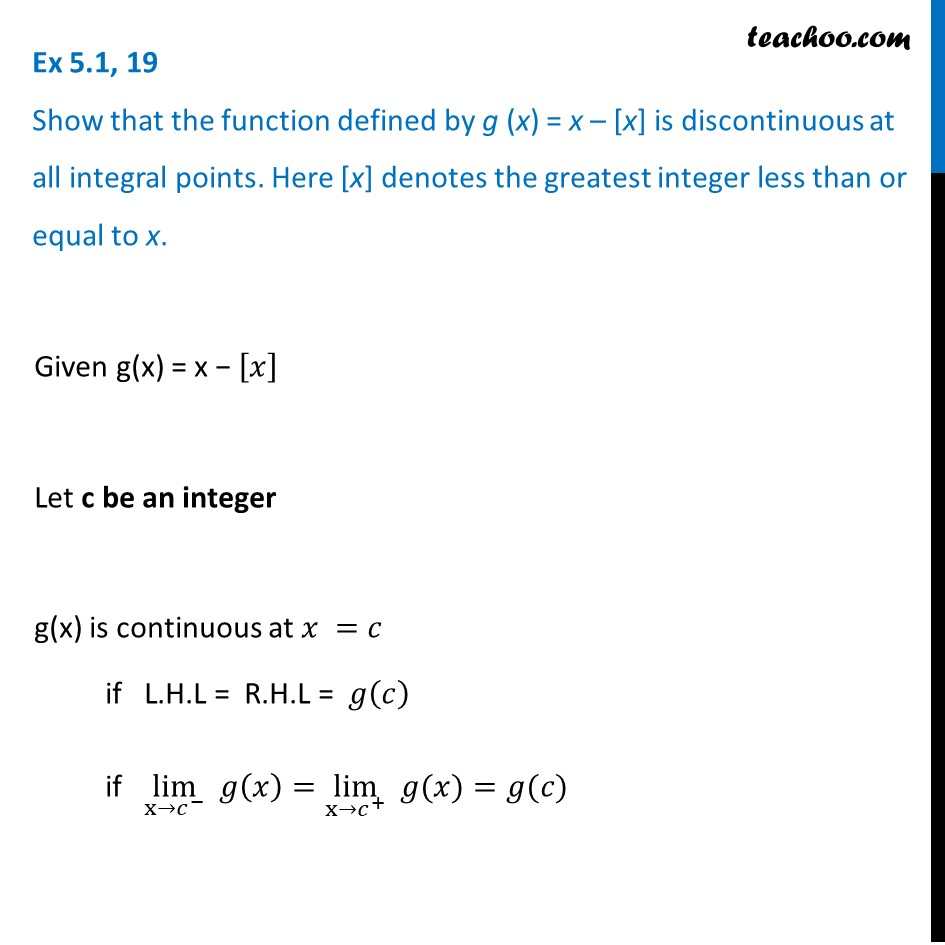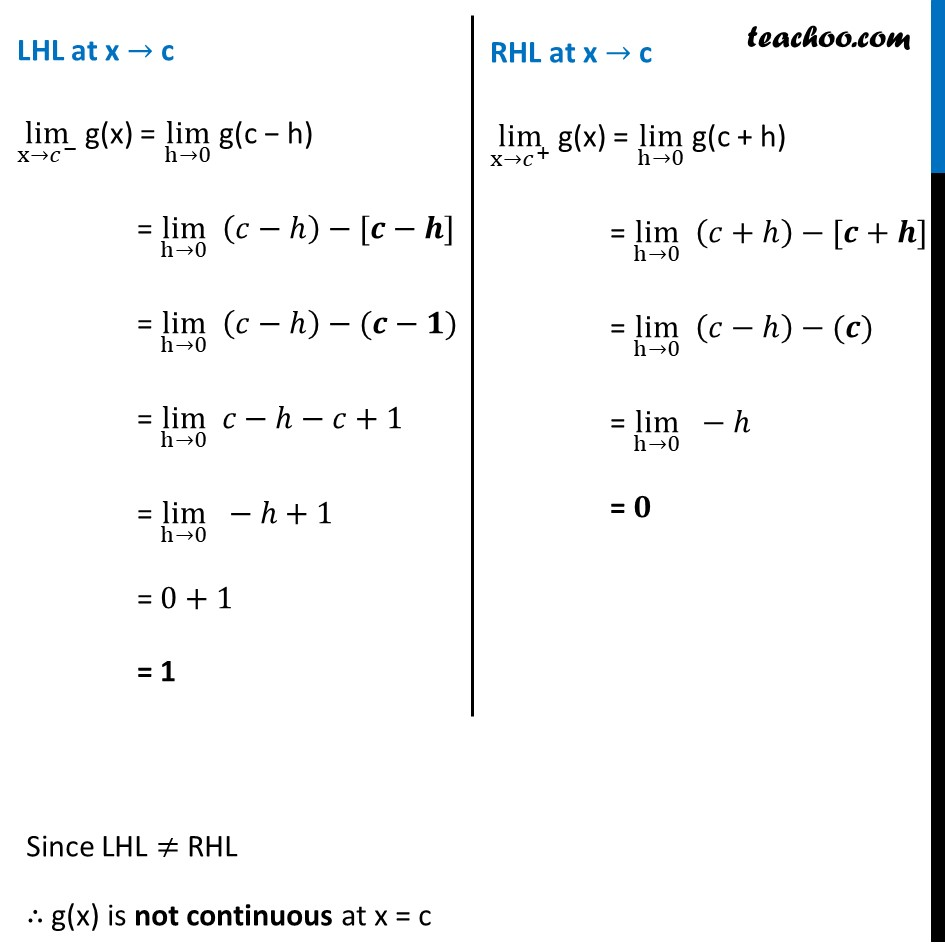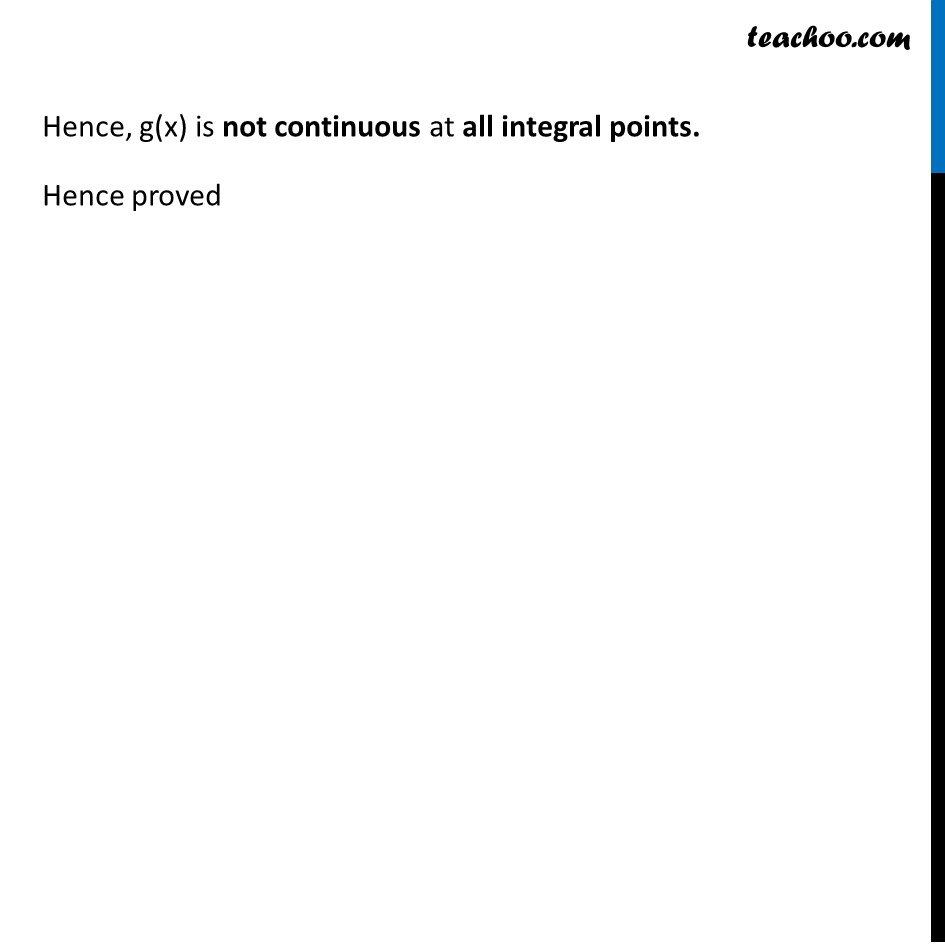Algebra of continous functions

Chapter 5 Class 12 Continuity and Differentiability (Term 1)
Concept wise### Transcript

Ex 5.1, 19 (Introduction) Show that the function defined by g (x) = x – [x] is discontinuous at all integral points. Here [x] denotes the greatest integer less than or equal to x. Ex 5.1, 19 Show that the function defined by g (x) = x – [x] is discontinuous at all integral points. Here [x] denotes the greatest integer less than or equal to x. Given g(x) = x − [𝑥] Let c be an integer g(x) is continuous at 𝑥 =𝑐 if L.H.L = R.H.L = 𝑔(𝑐) if lim┬(x→𝑐^− ) 𝑔(𝑥)=lim┬(x→𝑐^+ ) " " 𝑔(𝑥)=𝑔(𝑐) LHL at x → c lim┬(x→𝑐^− ) g(x) = lim┬(h→0) g(c − h) = lim┬(h→0) (𝑐−ℎ)−[𝒄−𝒉] = lim┬(h→0) (𝑐−ℎ)−(𝒄−𝟏) = lim┬(h→0) 𝑐−ℎ−𝑐+1 = lim┬(h→0) −ℎ+1 = 0+1 = 1 RHL at x → c lim┬(x→𝑐^+ ) g(x) = lim┬(h→0) g(c + h) = lim┬(h→0) (𝑐+ℎ)−[𝒄+𝒉] = lim┬(h→0) (𝑐−ℎ)−(𝒄) = lim┬(h→0) −ℎ = 𝟎 Since LHL ≠ RHL ∴ g(x) is not continuous at x = c Hence, g(x) is not continuous at all integral points. Hence proved